### python 数据挖掘算法

1、首先简述数据挖掘的过程

可以通过业务原始数据、公开的数据集、也可通过爬虫的方式获取。

数据极可能有噪音，不完整等缺陷，需要对数据进行数据标准化，方法有min-max 标准化， z-score 标准化，修正的标准z-score。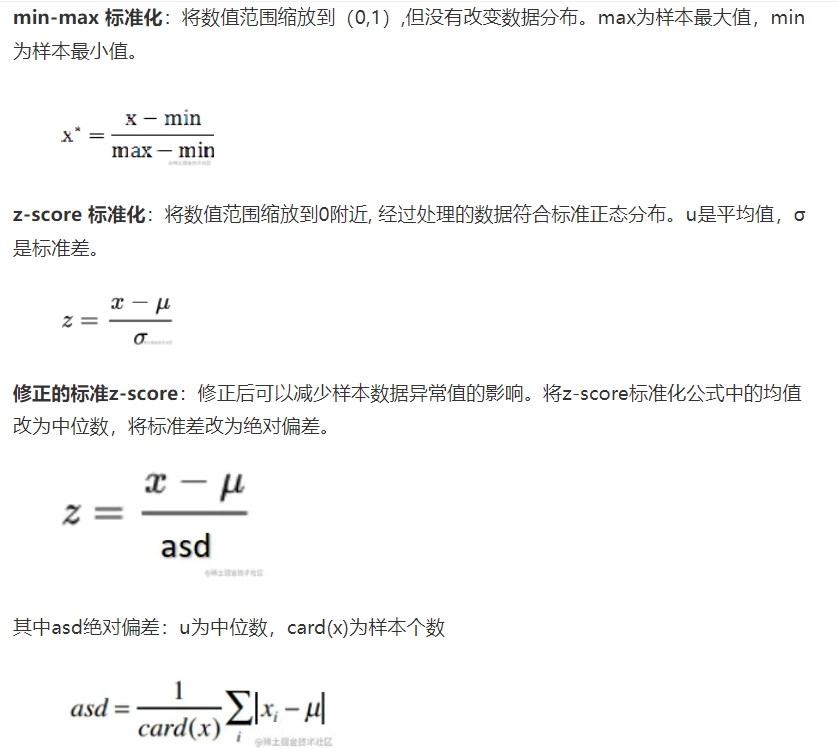将数据提取特征使这些数据符合特定数据挖掘算法的分析模型。数据模型有很多，等下详细讲解。

选择好的数据挖掘算法对数据进行训练

有两种主流方法：

十折交叉验证：将数据集随机分割成十个等份，每次用9份数据做训练集，1份数据做测试集，如此迭代10次。十折交叉验证的关键在于较平均地分为10份。

N折交叉验证又称为留一法：用几乎所有的数据进行训练，然后留一个数据进行测试，并迭代每一数据测试。留一法的优点是：确定性。

使用训练好的模型对数据进行预测。

对数据挖掘后的信息加以分析解释，并应用于实际的工作领域。

2、主要的算法模型讲解 ——基于sklearn

1）线性回归：希望所有点都落在直线上，所有点离直线的距离最近。首先假设好y=ax+b中a和b的值，然后计算每个数据点到这条直线上的距离总和，目的是要使这个总和最小！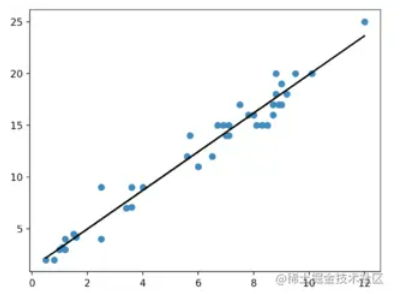``from sklearn.linear_model import LinearRegression# 定义线性回归模型model = LinearRegression(fit_intercept=True, normalize=False,     copy_X=True, n_jobs=1)"""参数---    fit_intercept：是否计算截距。False-模型没有截距    normalize： 当fit_intercept设置为False时，该参数将被忽略。 如果为真，则回归前的回归系数X将通过减去平均值并除以l2-范数而归一化。     n_jobs：指定线程数"""``

2）逻辑回归：二分算法，用于两分类问题。需要预测函数的“大概形式”， 比如是线性还是非线性的。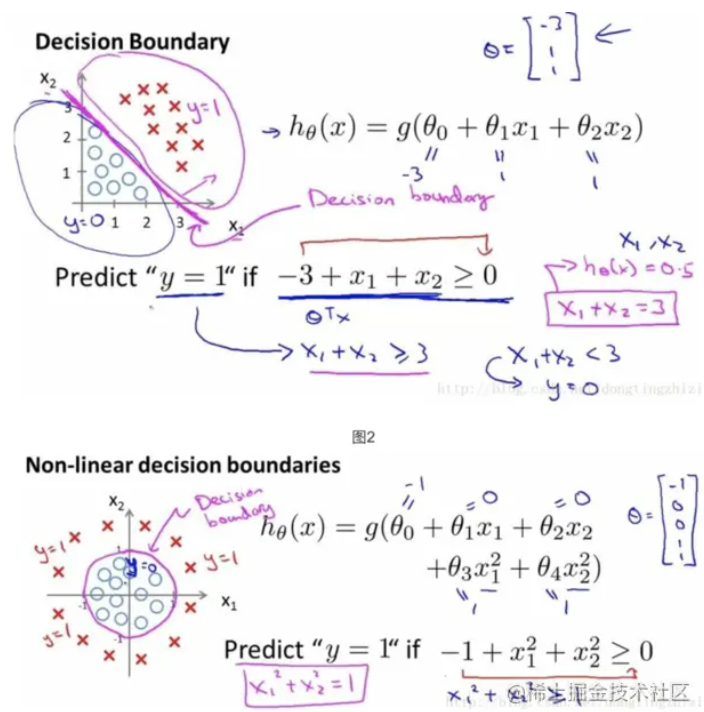``from sklearn.linear_model import LogisticRegression# 定义逻辑回归模型model = LogisticRegression(penalty=’l2’, dual=False, tol=0.0001, C=1.0,     fit_intercept=True, intercept_scaling=1, class_weight=None,     random_state=None, solver=’liblinear’, max_iter=100, multi_class=’ovr’,     verbose=0, warm_start=False, n_jobs=1) """参数---    penalty：使用指定正则化项（默认：l2）    dual: n_samples > n_features取False（默认）    C：正则化强度的反，值越小正则化强度越大    n_jobs: 指定线程数    random_state：随机数生成器    fit_intercept: 是否需要常量"""``

3）朴素贝叶斯算法NB：用于判断某件事的发生概率，我就曾用此算法做过舆情分类器。将一些语句变为01二维矩阵，计算词语的出现频率，从而判断语句的情感色彩是怎样的。

``from sklearn import naive_bayesmodel = naive_bayes.GaussianNB() # 高斯贝叶斯model = naive_bayes.MultinomialNB(alpha=1.0, fit_prior=True, class_prior=None)model = naive_bayes.BernoulliNB(alpha=1.0, binarize=0.0, fit_prior=True, class_prior=None)"""文本分类问题常用MultinomialNB参数---    alpha：平滑参数    fit_prior：是否要学习类的先验概率；false-使用统一的先验概率    class_prior: 是否指定类的先验概率；若指定则不能根据参数调整    binarize: 二值化的阈值，若为None，则假设输入由二进制向量组成"""``

4）决策树DT：类似流程图的树结构，它使用分支方法来说明决策的每个可能结果。树中的每个节点代表对特定变量的测试 – 每个分支都是该测试的结果。

tree.DecisionTreeClassifier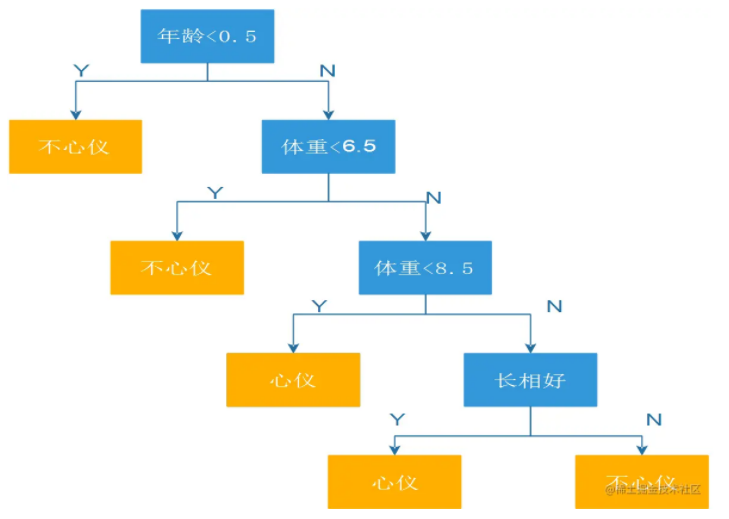``from sklearn import tree model = tree.DecisionTreeClassifier(criterion=’gini’, max_depth=None,     min_samples_split=2, min_samples_leaf=1, min_weight_fraction_leaf=0.0,     max_features=None, random_state=None, max_leaf_nodes=None,     min_impurity_decrease=0.0, min_impurity_split=None,     class_weight=None, presort=False)"""参数---    criterion ：特征选择准则gini/entropy    max_depth：树的最大深度，None-尽量下分    min_samples_split：分裂内部节点，所需要的最小样本树    min_samples_leaf：叶子节点所需要的最小样本数    max_features: 寻找最优分割点时的最大特征数    max_leaf_nodes：优先增长到最大叶子节点数    min_impurity_decrease：如果这种分离导致杂质的减少大于或等于这个值，则节点将被拆分。"""``

5）支持向量机SVM：就是判断线性可分不可分，能不能用直线分割两类数据！理论可以推广到三维，甚至思维以上的特征空间。三维使用平面来分隔数据，四维和四维以上因为人类 无法直观的感知出来，所以画不出来，但是能分隔数据，存在这样的平面叫做超平面。

SVC 二值分类器 工作原理_lipeitong333的博客-CSDN博客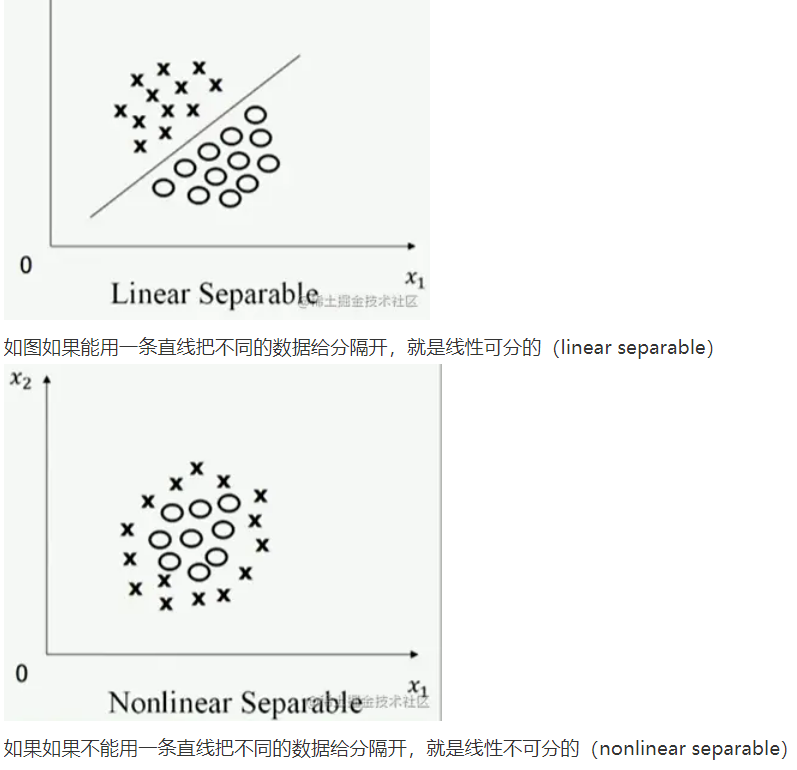``from sklearn.svm import SVCmodel = SVC(C=1.0, kernel=’rbf’, gamma=’auto’)"""参数---    C：误差项的惩罚参数C    gamma: 核相关系数。浮点数，If gamma is ‘auto’ then 1/n_features will be used instead."""``

6）k近邻算法KNN：采用测量不同特征值之间距离的方法对数据进行分类的一个算法。

K近邻算法，即是给定一个训练数据集，对新的输入实例，在训练数据集中找到与该实例最邻近的K个实例，这K个实例的多数属于某个类，就把该输入实例分类到这个类中。（这就类似于现实生活中少数服从多数的思想）根据这个说法，咱们来看下引自维基百科上的一幅图：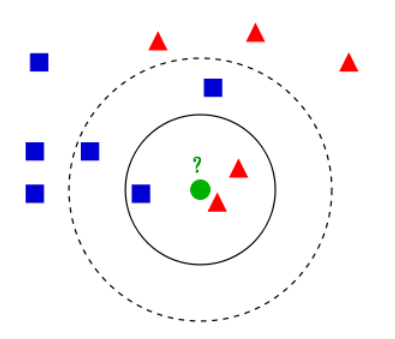• 如果K=3，绿色圆点的最邻近的3个点是2个红色小三角形和1个蓝色小正方形，少数从属于多数，基于统计的方法，判定绿色的这个待分类点属于红色的三角形一类。
•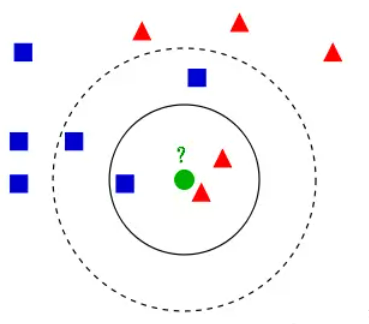• 如果K=5，绿色圆点的最邻近的5个邻居是2个红色三角形和3个蓝色的正方形，还是少数从属于多数，基于统计的方法，判定绿色的这个待分类点属于蓝色的正方形一类。
``from sklearn import neighbors#定义kNN分类模型model = neighbors.KNeighborsClassifier(n_neighbors=5, n_jobs=1) # 分类model = neighbors.KNeighborsRegressor(n_neighbors=5, n_jobs=1) # 回归"""参数---    n_neighbors： 使用邻居的数目    n_jobs：并行任务数"""``

7）K-均值聚类（K-means）

• 定义目标聚类数K，例如，k=3
• 随机初始化的 k 个聚类中心(controids)
• 计算每个数据点到K个聚类中心的Euclidean Distance，然后将数据点分到Euclidean Distance最小的对应类聚中心的那类
• 针对每个类别，重新计算它的聚类中心；
• 重复上面 3-4 两步操作，直到达到某个中止条件（迭代次数、最小误差变化等）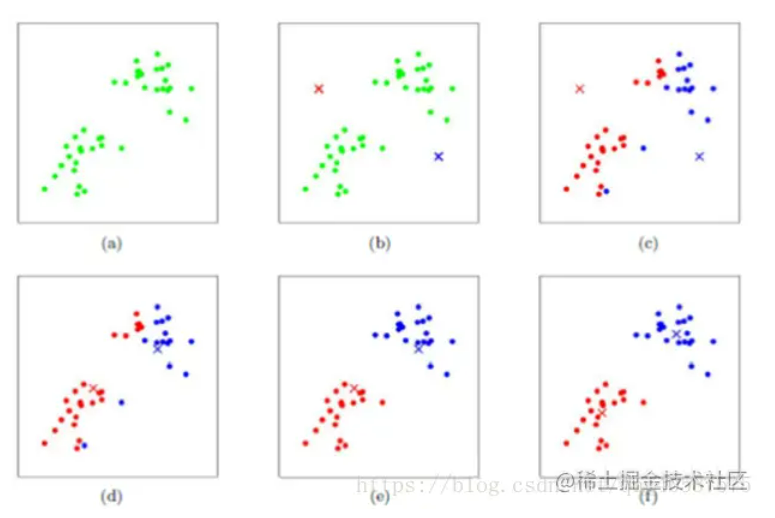``import pandas as pdimport matplotlib.pyplot as pltfrom sklearn.cluster import KMeans df = pd.DataFrame({"x": [25, 34, 22, 27, 33, 33, 31, 22, 35, 34, 67, 54, 57, 43, 50, 57, 59, 52, 65, 47, 49, 48, 35, 33, 44, 45, 38, 43, 51, 46],                   "y": [79, 51, 53, 78, 59, 74, 73, 57, 69, 75, 51, 32, 40, 47, 53, 36, 35, 59, 59, 50, 25, 20, 14, 12, 20, 5,  29, 27, 8,  7]}) kmeans = KMeans(n_clusters=3).fit(df)centroids = kmeans.cluster_centers_# 打印类聚中心print(type(centroids), centroids) # 可视化类聚结果fig, ax = plt.subplots()ax.scatter(df['x'],df['y'],c=kmeans.labels_.astype(float),s=50, alpha=0.5)ax.scatter(centroids[:, 0], centroids[:, 1], c='red', s=50)plt.show()``

More：

FP-Growth_lipeitong333的博客-CSDN博客

Apriori关联分析算法 -尿布与啤酒的故事_lipeitong333的博客-CSDN博客

ps）：我们在机器学习中一直会遇到两种问题，一种是回归问题，一种是分类问题。我们从字面上理解，很容易知道分类问题其实是将我们现有的数据分成若干类，然后对于新的数据，我们根据所分得类而进行划分；而回归问题是将现有数据拟合成一条函数，根据所拟合的函数来预测新的数据。 这两者的区别就在于输出变量的类型。回归是定量输出，或者说是预测连续变量；分类问题书定量输出，预测离散变量。Po一张我在知乎上看到的一张图片，解释的很好：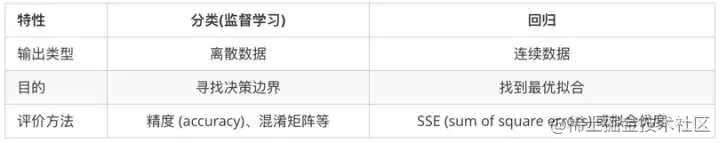3、sklearn自带方法joblib来进行保存训练好的模型

``from sklearn.externals import joblib # 保存模型joblib.dump(model, 'model.pickle') #载入模型model = joblib.load('model.pickle')``

https://juejin.cn/post/6844903513504530446、https://juejin.cn/post/6974596282694254606、机器学习神器：sklearn的快速使用 – 掘金 (juejin.cn)、机器学习之逻辑回归(纯python实现) – 掘金 (juejin.cn)、